# GMAT Math : Prisms

## Example Questions

← Previous 1

### Example Question #1 : Dsq: Calculating The Surface Area Of A Prism

What is the volume of a cardboard box with six rectangular surfaces?

Statement 1: The length and width are each half the height.

Statement 2: The height is ten inches longer than the width.

EITHER statement ALONE is sufficient to answer the question.

Statement 2 ALONE is sufficient to answer the question, but Statement 1 ALONE is NOT sufficient to answer the question.

BOTH statements TOGETHER are insufficient to answer the question.

BOTH statements TOGETHER are sufficient to answer the question, but NEITHER statement ALONE is sufficient to answer the question.

Statement 1 ALONE is sufficient to answer the question, but Statement 2 ALONE is NOT sufficient to answer the question.

BOTH statements TOGETHER are sufficient to answer the question, but NEITHER statement ALONE is sufficient to answer the question.

Explanation:

To know the volume of the box, which is shaped like a rectangular prism, you multiply the length, the width, and the height together. Neither statement alone gives you those dimensions, just clues as to how they are related. But together, you can form a system of linear equations using height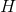and width (and length)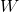.

By the first and second statements, respectively,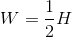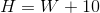This system can be solved: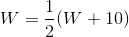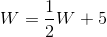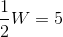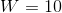From this, you can determine the length and height, and, subsequently, the volume.

### Example Question #2 : Dsq: Calculating The Surface Area Of A Prism

A box with equal length, width, and height has the following dimensions.

I) The diagonal of the cube is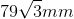.

II) The volume of the cube is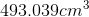.

What is the surface area of the box?

Statement II is sufficient to answer the question, but statement I is not sufficient to answer the question.

Either statement is sufficient to answer the question.

Both statements are needed to answer the question.

Statement I is sufficient to answer the question, but statement II is not sufficient to answer the question.

Either statement is sufficient to answer the question.

Explanation:

To find surface area, we need to know the side length of the box.

We are told the box has equal length, width and height. This means it is a cube.

I) Gives us the diagonal of the cube, from this we can find the side length.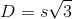, where s is the side length.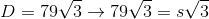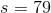Now we can use the side length to find the surface area of the box.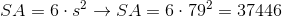II) Gives us the volume of the cube, from which we can also find the side length.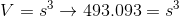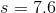From here we can find the surface area.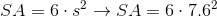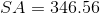Thus, either statement is sufficient.

### Example Question #3 : Dsq: Calculating The Surface Area Of A Prism

Fry recently met a robot known for bending things. The robot wants to make a box out of steel by bending a single sheet of metal. Find the total area of the sheet of metal given the following:

I) The box will be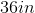long.

II) The box's height will be 2 feet taller than its length, and the box's width will be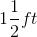less than its length.

Statement II is sufficient to answer the question, but statement I is not sufficient to answer the question.

Both statements are needed to answer the question.

Either statement is sufficient to answer the question.

Statement I is sufficient to answer the question, but statement II is not sufficient to answer the question.

Both statements are needed to answer the question.

Explanation:

Fry recently met a robot known for bending things. The robot wants to make a box out of steel by bending a single sheet of metal. Find the total area of the sheet of metal given the following:

I) The box will belong

II) The box's height will be 2 feet taller than its length, and the box's width will beless than its length.

This question is a surface area question in disguise. To find the surface area of a prism, we need the area of each side. Begin by using I and II to find each side length:

Length: 36 inches or 3 feet

Height: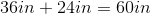or 5 feet

Width: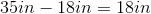or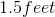So, we have all our side lengths. Next, use the following formula for surface area of a rectangular prism: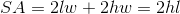Where l,w, and h are length, width and height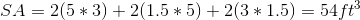### Example Question #71 : Rectangular Solids & Cylinders

Given a right square pyramid and a right cone, which, if either, has greater volume?

Statement 1: The two have the same height.

Statement 2: The base of the cone and the base of the pyramid have the same area.

EITHER statement ALONE is sufficient to answer the question.

BOTH statements TOGETHER are insufficient to answer the question.

Statement 2 ALONE is sufficient to answer the question, but Statement 1 ALONE is NOT sufficient to answer the question.

BOTH statements TOGETHER are sufficient to answer the question, but NEITHER statement ALONE is sufficient to answer the question.

Statement 1 ALONE is sufficient to answer the question, but Statement 2 ALONE is NOT sufficient to answer the question.

BOTH statements TOGETHER are sufficient to answer the question, but NEITHER statement ALONE is sufficient to answer the question.

Explanation:

Given base areaheight, the volume of either a pyramid or a cone is equal to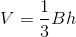.

If we know the heights of the two figures are the same, we still need to know which has the greater base area in order to know which has the greater volume, so Statement 1 is insufficient. By a similar argument, Statement 2 is insufficient.

If we know both heights are equal and that both bases have equal area, then we know that the volumes are equal.

### Example Question #72 : Rectangular Solids & Cylinders

Find the volume of a rectangular prism.

Statement 1: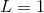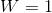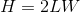Statement 2:  The diagonal is 6.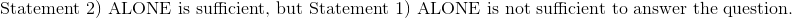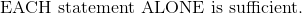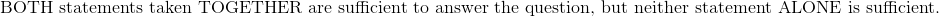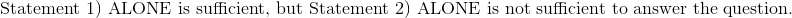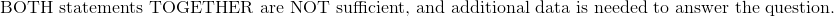Explanation:

Statement 1:The height can be easily solved for by substituting the length and width. Height is 2, and the volume can be calculated by the following formula.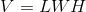Statement 2:  The diagonal is 6.

Write the equation of the diagonal in terms of length, width, and height.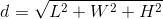Although the lengths from Statement 1 will yield a diagonal of six, there are multiple combinations of length, width, and height which will also give a diagonal of six.  The product of these three dimensions may or may not give a volume of six.  Statement 2 has insufficient information to calculate the volume.

Therefore:### Example Question #73 : Rectangular Solids & Cylinders

While deep-sea diving, Al found a rectangular treasure chest. Before he opens it, he wants to find the volume of the chest. What is it?

I) Al measured the chest's longest side to be twice its smallest side and one-and-a-half times the length of the medium side.

II) Al found that the chest's shortest side is 2.5ft long.

Statement I is sufficient to answer the question, but Statement II is not sufficient to answer the question.

Statement II is sufficient to answer the question, but Statement I is not sufficient to answer the question.

Either statement is sufficient to answer the question.

Both statements are needed to answer the question.

Both statements are needed to answer the question.

Explanation:

To find the volume of a rectangular solid, use the following formula: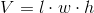Statement I relates the length, width and height of the treasure chest.

Statement II gives us the length of the shortest side of the treasure chest.

Use Statement II and the relationships described in Statement I to find each side, then multiply them all together to get your answer.

If the shortest side is 2.5ft, then the longest side must be 5ft.

If the longest side is 5ft, then the medium side can be found via the following: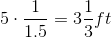So the volume is as follows: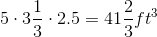### Example Question #74 : Rectangular Solids & Cylinders

Find the length of the diagonal of cube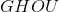.

I)has a volume of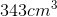.

II)has a surface area of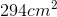.

Statement II is sufficient to answer the question, but statement I is not sufficient to answer the question.

Statement I is sufficient to answer the question, but statement II is not sufficient to answer the question.

Either statement is sufficient to answer the question.

Both statements are needed to answer the question.

Either statement is sufficient to answer the question.

Explanation:

To find the length of the diagonal, we need the side length.

I) Gives us the volume of the cube. Take the cubed root to find the side length.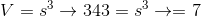II) Gives us the surface area, divide by 6 (the number of faces in a cube) and take the square root to find the side length.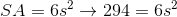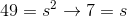Diagonal of a cube is the side length times the square root of three. Alternatively, this can be found using the Pythagorean Theorem twice.

Either way, we can use I) or II) to find our side lenght and then our diagonal.

### Example Question #75 : Rectangular Solids & Cylinders

What is the diagonal of a rectangular prism?

1) Its surface area is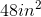2) Its height = twice its width = thrice its length.

Statement 2 alone is sufficient.

Neither of the statements, separate or together, is sufficient.

Together, the two statements are sufficient.

Statement 1 alone is sufficient.

Either of the statements is sufficient.

Together, the two statements are sufficient.

Explanation:

The diagonal of a rectangular prism is found via the formulaThe second statement reduces this to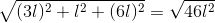However, the actual length is unknown. Statement 1 allows the calculation of a numerical value: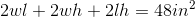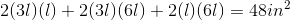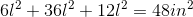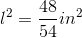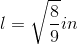### Example Question #76 : Rectangular Solids & Cylinders

A carpenter is building a box to hold his tools. Find the legth of the second smallest side of the box.

I) The box will have a volume of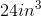.

II) The smallest side is half the length of the longest side and the middle side is three-quarters of the length of the longest side.

Statement I is sufficient to answer the question, but statement II is not sufficient to answer the question.

Either statement is sufficient to answer the question.

Statement II is sufficient to answer the question, but statement I is not sufficient to answer the question.

Both statements are needed to answer the question.

Both statements are needed to answer the question.

Explanation:

Volume of a prism is found by: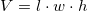We are given the volume in statement I.

We are told how the sides relate in statement II.

Put together these two statements will allow us to set up an equation to find the middle side.

### Example Question #77 : Rectangular Solids & Cylinders

A pirate wants to hide all of his treasure. He commisions a local woodworker to build him a series of wooden chests of volume of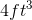. Find the length of the longest side given the following:

I) The shortest side will be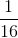the length of the medium side.

II) The middle side will be 2 feet long.

Both statements are needed to answer the question.

Either statement is sufficient to answer the question.

Statement I is sufficient to answer the question, but statement II is not sufficient to answer the question.

Statement II is sufficient to answer the question, but statement I is not sufficient to answer the question.

Both statements are needed to answer the question.

Explanation:

A pirate wants to hide all of his treasure. He commisions a local woodworker to build him a series of wooden chests of volume of. Find the length of the longest side given the following:

I) The shortest side will bethe length of the medium side

II) The middle side will be 2 feet long

Use I) and II) to find the length of the smallest side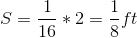Next, use the short and medium sides, along with info in the prompt, to find the last side: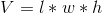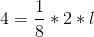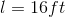← Previous 1

### All GMAT Math Resources